﻿ 纳米尺度下半空间内浅埋圆形弹性夹杂对SH波的散射 Scattering of a Nanoscale Circular Elastic Inclusion on SH Wave in a Semi-Space

International Journal of Mechanics Research
Vol.07 No.02(2018), Article ID:25508,9 pages
10.12677/IJM.2018.72008

Scattering of a Nanoscale Circular Elastic Inclusion on SH Wave in a Semi-Space

Zhiying Ou, Yanbin Zheng

School of Science, Lanzhou University of Technology, Lanzhou Gansu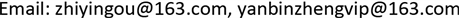Received: Jun. 1st, 2018; accepted: Jun. 14th, 2018; published: Jun. 21st, 2018ABSTRACT

Using complex function method, multi-polar coordinate method, image-source method, and Graf’s addition formula, the scattering of SH wave by a nano-circular inclusion in elastic half space was studied. Firstly, according to the boundary conditions, the reflected, scattered and refracted wave functions in medium were calculated. Then the wave functions of the corresponding stress field in complex coordinate system were derived. Finally, the calculation examples and results of the dynamic stress concentration around the interface of the circular inclusion were presented in the paper. The variation of circumferential dynamic stress around the boundary of circular inclusion with dimensionless wave number and the change of incidence angle were discussed in detail. The researcher also analyzed the influence of surface parameters on dynamic stress concentration and compared the dynamic stress concentration under macroscopic and microscopic conditions. The results show that the stress concentration at the boundary of the circular inclusion is stronger with the smaller the surface parameter and the higher the dimensionless wave number.

Keywords:Circular Inclusion, Complex Function Method, Dynamic Stress Concentration1. 引言

2. 问题模型和分析

${\nabla }^{2}W+{K}^{2}W=0$ (1)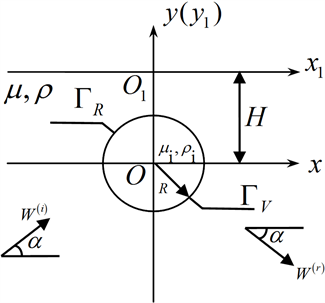Figure 1. Scattering of a shallow buried circular inclusion in a half space for a steady incident plane SH wave

$\left\{\begin{array}{l}{\tau }_{rz}=\mu \left(\frac{\partial W}{\partial z}{\text{e}}^{i\theta }+\frac{\partial W}{\partial \stackrel{¯}{z}}{\text{e}}^{-i\theta }\right)\\ {\tau }_{\theta z}=i\mu \left(\frac{\partial W}{\partial z}{\text{e}}^{i\theta }-\frac{\partial W}{\partial \stackrel{¯}{z}}{\text{e}}^{-i\theta }\right)\end{array}$ (2)

${W}^{\left(\text{I}\right)}={W}_{0}{\text{e}}^{\frac{ik}{2}\left[\left(z{\text{e}}^{-i\alpha }+\stackrel{¯}{z}{\text{e}}^{i\alpha }\right)\right]{\text{e}}^{-iHk\mathrm{sin}\alpha }}$ (3)

${W}^{\left(\text{r}\right)}={W}_{0}{\text{e}}^{\frac{ik}{2}\left[\left(z{\text{e}}^{-i\alpha }+\stackrel{¯}{z}{\text{e}}^{i\alpha }\right)\right]{\text{e}}^{iHk\mathrm{sin}\alpha }}$ (4)

${W}^{\left(\text{s}\right)}=\underset{n=-\infty }{\overset{\infty }{\sum }}{A}_{n}\left\{{H}_{n}^{\left(1\right)}\left(k|z|\right){\left(\frac{z}{|z|}\right)}^{n}+{H}_{n}^{\left(1\right)}\left(k|z-2Hi|\right){\left(\frac{z-2Hi}{|z-2Hi|}\right)}^{-n}\right\}$ (5)

${W}^{\left(\text{f}\right)}=\underset{n=-\infty }{\overset{\infty }{\sum }}{B}_{n}{J}_{n}\left({k}_{i}|z|\right){\left(\frac{z}{|z|}\right)}^{n}$ (6)

${\tau }_{rz}^{\left(\text{I}\right)}=\frac{\text{i}k\mu {W}_{0}}{2}\left[{\text{e}}^{i\left(\theta -\alpha \right)}+{\text{e}}^{-i\left(\theta -\alpha \right)}\right]{\text{e}}^{-iHk\mathrm{sin}\left(\alpha \right)}{\text{e}}^{\frac{ik}{2}\left(z{\text{e}}^{-i\alpha }+\stackrel{¯}{z}{\text{e}}^{i\alpha }\right)}$ (7)

${\tau }_{\theta \text{z}}^{\left(\text{I}\right)}=\frac{-k\mu {W}_{0}}{2}\left[{\text{e}}^{i\left(\theta -\alpha \right)}+{\text{e}}^{-i\left(\theta -\alpha \right)}\right]{\text{e}}^{-iHk\mathrm{sin}\left(\alpha \right)}{\text{e}}^{\frac{ik}{2}\left(z{\text{e}}^{-i\alpha }+\stackrel{¯}{z}{\text{e}}^{i\alpha }\right)}$ (8)

${\tau }_{rz}^{\left(\text{r}\right)}=\frac{\text{i}k\mu {W}_{0}}{2}\left[{\text{e}}^{i\left(\theta \text{+}\alpha \right)}+{\text{e}}^{-i\left(\theta \text{+}\alpha \right)}\right]{\text{e}}^{iHk\mathrm{sin}\left(\alpha \right)}{\text{e}}^{\frac{ik}{2}\left(z{\text{e}}^{i\alpha }+\stackrel{¯}{z}{\text{e}}^{-i\alpha }\right)}$ (9)

${\tau }_{\theta z}^{\left(\text{r}\right)}=\frac{-k\mu {W}_{0}}{2}\left[{\text{e}}^{i\left(\theta \text{+}\alpha \right)}-{\text{e}}^{-i\left(\theta \text{+}\alpha \right)}\right]{\text{e}}^{iHk\mathrm{sin}\left(\alpha \right)}{\text{e}}^{\frac{ik}{2}\left(z{\text{e}}^{i\alpha }+\stackrel{¯}{z}{\text{e}}^{-i\alpha }\right)}$ (10)

$\begin{array}{c}{\tau }_{rz}^{\left(\text{s}\right)}=\frac{k\mu }{2}\underset{n=-\infty }{\overset{\infty }{\sum }}{A}_{n}\left[{H}_{n-1}^{\left(1\right)}\left(k|z|\right){\left(\frac{z}{|z|}\right)}^{n\text{-}1}{\text{e}}^{i\theta }-{H}_{n\text{+}1}^{\left(1\right)}\left(k|z|\right){\left(\frac{z}{|z|}\right)}^{n+1}{\text{e}}^{-i\theta }\\ \text{\hspace{0.17em}}\text{\hspace{0.17em}}-{H}_{n+1}^{\left(1\right)}\left(k|z-2Hi|\right){\left(\frac{z-2Hi}{|z-2Hi|}\right)}^{-n-1}{\text{e}}^{i\theta }+{H}_{n-1}^{\left(1\right)}\left(k|z-2Hi|\right){\left(\frac{z-2Hi}{|z-2Hi|}\right)}^{-n+1}{\text{e}}^{-i\theta }\right]\end{array}$ (11)

$\begin{array}{c}{\tau }_{\theta z}^{\left(\text{s}\right)}=\frac{\text{i}k\mu }{2}\underset{n=-\infty }{\overset{\infty }{\sum }}{A}_{n}\left[{H}_{n-1}^{\left(1\right)}\left(k|z|\right){\left(\frac{z}{|z|}\right)}^{n\text{-}1}{\text{e}}^{i\theta }+{H}_{n\text{+}1}^{\left(1\right)}\left(k|z|\right){\left(\frac{z}{|z|}\right)}^{n+1}{\text{e}}^{-i\theta }\\ \text{\hspace{0.17em}}\text{\hspace{0.17em}}-{H}_{n+1}^{\left(1\right)}\left(k|z-2Hi|\right){\left(\frac{z-2Hi}{|z-2Hi|}\right)}^{-n-1}{\text{e}}^{i\theta }-{H}_{n-1}^{\left(1\right)}\left(k|z-2Hi|\right){\left(\frac{z-2Hi}{|z-2Hi|}\right)}^{-n+1}{\text{e}}^{-i\theta }\right]\end{array}$ (12)

${\tau }_{rz}^{\left(\text{f}\right)}=\frac{{k}_{i}{\mu }_{i}}{2}\underset{n=-\infty }{\overset{\infty }{\sum }}{B}_{n}\left[{J}_{n-1}\left({k}_{i}|z|\right){\left(\frac{z}{|z|}\right)}^{n}+{J}_{n+1}\left({k}_{i}|z|\right){\left(\frac{z}{|z|}\right)}^{n}\right]$ (13)

${W}^{\left({\text{t}}_{1}\right)}={W}^{\left(\text{I}\right)}+{W}^{\left(\text{r}\right)}+{W}^{\left(\text{s}\right)}$ (14)

${W}^{\left({\text{t}}_{2}\right)}={W}^{\left(\text{f}\right)}$ (15)

${\tau }_{rz}^{\left({\text{t}}_{1}\right)}={\tau }_{rz}^{\left(\text{I}\right)}+{\tau }_{rz}^{\left(\text{r}\right)}+{\tau }_{rz}^{\left(\text{s}\right)}$ (16)

${\tau }_{\theta z}^{\left({\text{t}}_{1}\right)}={\tau }_{\theta z}^{\left(\text{I}\right)}+{\tau }_{\theta z}^{\left(\text{r}\right)}+{\tau }_{\theta z}^{\left(\text{s}\right)}$ (17)

${\tau }_{rz}^{\left({\text{t}}_{2}\right)}={\tau }_{rz}^{\left(\text{f}\right)}$ (18)

$\left\{\begin{array}{l}{\Gamma }_{H}:{\tau }_{yz}^{{\text{t}}_{1}}\left(x,H\right)=0\\ {\Gamma }_{R}:{\tau }_{rz}^{{\text{t}}_{1}}\left(R,\theta \right)-{\tau }_{rz}^{{\text{t}}_{2}}\left(R,\theta \right)=-S\frac{\partial {\tau }_{\theta z}^{{\text{t}}_{1}}}{\partial \theta }\\ {\Gamma }_{R}:{W}^{\left({\text{t}}_{1}\right)}\left(R,\theta \right)={W}^{\left({\text{t}}_{2}\right)}\left(R,\theta \right)\end{array}$ (19)

$\left\{\begin{array}{l}\underset{n=-\infty }{\overset{\infty }{\sum }}{a}_{11}{B}_{n}+{a}_{12}{A}_{n}={b}_{1}\\ \underset{n=-\infty }{\overset{\infty }{\sum }}{a}_{21}{B}_{n}+{a}_{22}{A}_{n}={b}_{2}\end{array}$ (20)

$\left\{\begin{array}{l}\underset{n=-\infty }{\overset{\infty }{\sum }}{c}_{11}{B}_{n}+{c}_{12}{A}_{n}={d}_{1}\\ \underset{n=-\infty }{\overset{\infty }{\sum }}{c}_{21}{B}_{n}+{c}_{22}{A}_{n}={d}_{2}\end{array}$ (21)

$\begin{array}{l}{c}_{jk}=\frac{1}{2\text{π}}{\int }_{-\text{π}}^{\text{π}}{a}_{jk}{\text{e}}^{-im\theta }\text{d}\theta \\ {d}_{j}=\frac{1}{2\text{π}}{\int }_{-\text{π}}^{\text{π}}{b}_{j}{\text{e}}^{-im\theta }\text{d}\theta \end{array}$ $\left(j,k=1,2\right)$ (22)

$\gamma =|\frac{{\tau }_{\theta \text{z}}^{{t}_{1}}}{ku{W}_{0}^{\left(1\right)}}|$ (23)

3. 算例及分析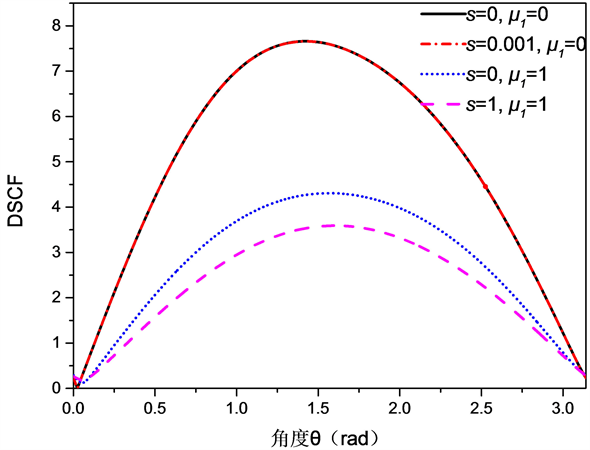Figure 2. The ring direction angle distribution of the cyclic dynamic stress concentration coefficient at the boundary of the circular inclusion (hole) the level of incident wave kR = 2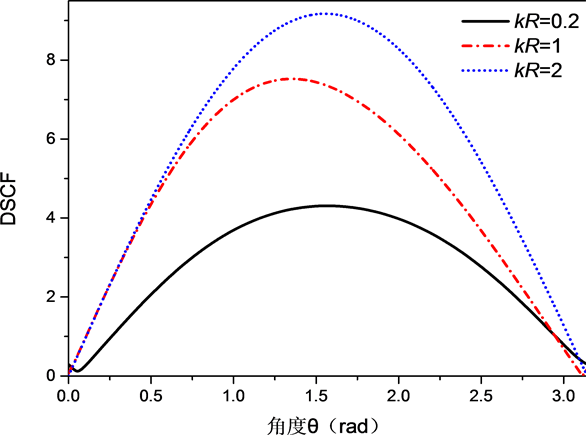Figure 3. The ring direction angle distribution of the cyclic dynamic stress concentration coefficient at the boundary of the circular inclusion at the incident wave level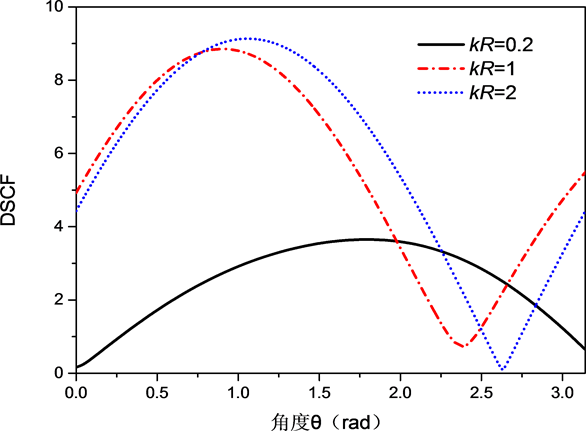Figure 4. The ring direction angle distribution of the cyclic dynamic stress concentration coefficient at the incident wave at $\frac{\text{π}}{3}$ rad of incidence at the boundary of the circular inclusion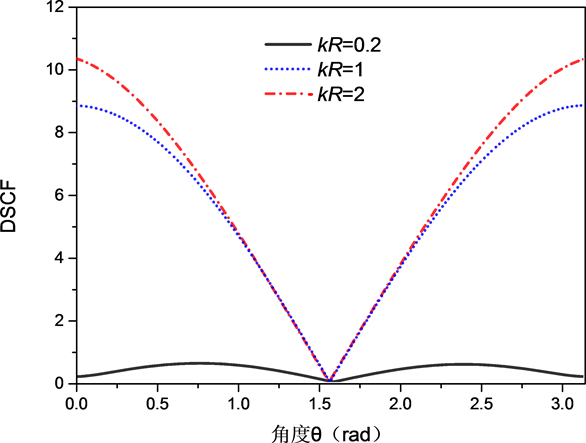Figure 5. Circular angle distribution of the cyclic dynamic stress concentration coefficient at the boundary of a circular inclusion in a vertical incident wave incident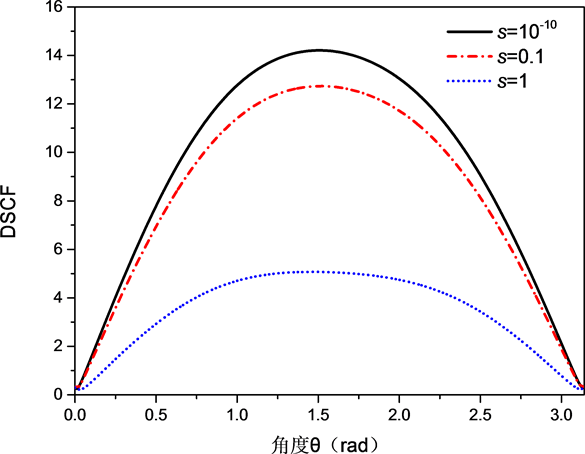Figure 6. The ring direction angle distribution of the cyclic dynamic stress concentration coefficient at the boundary of the circular inclusion at the incident wave level when the incident wave is incident at kR = 0.2, S = 10−10, 0.1, 1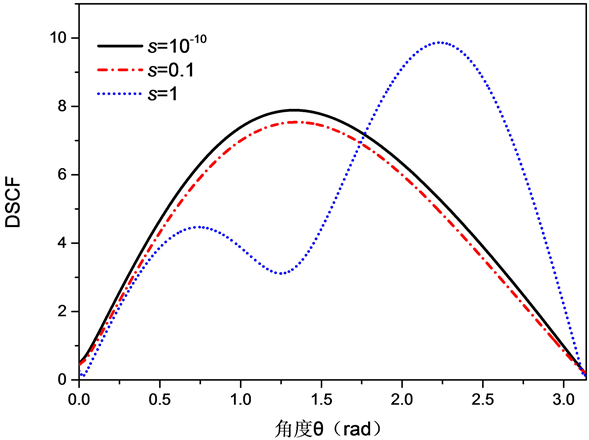Figure 7. The ring direction angle distribution of the cyclic dynamic stress concentration coefficient at the incident wave level when the incident wave is incident at the level of kR = 0.2, S = 10−10, 0.1, 1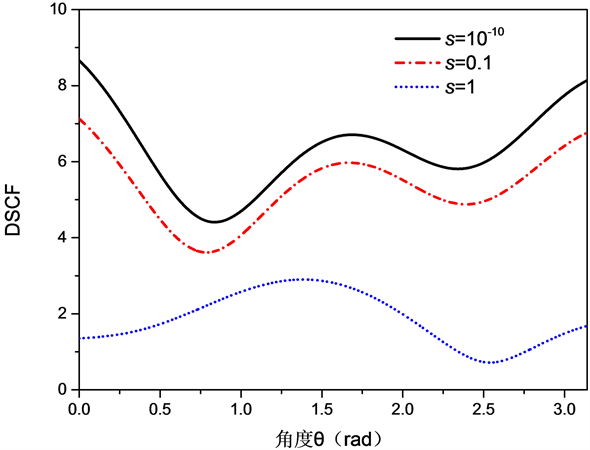Figure 8. When the incident wave is $kR=0.2$ , $S={10}^{-10},0.1,1$ the ring direction of the dynamic stress concentration coefficient is distributed at the boundary of the circular inclusion at the $\frac{\text{π}}{3}$ rad incident

4. 结论

1) 在纳米尺度下圆形夹杂边界处的应力集中程度显著，表面参数 $S$ 越小，引起的圆形夹杂边界处的应力集中程度越强。

2) 在纳米尺度下，无量纲波数 $kR$ 越大，引起的圆形夹杂边界处的应力集中程度越强，和宏观条件下一致。

Scattering of a Nanoscale Circular Elastic Inclusion on SH Wave in a Semi-Space[J]. 力学研究, 2018, 07(02): 66-74. https://doi.org/10.12677/IJM.2018.72008

1. 1. Pao, Y.H., Mow, C.C. and Achenbach, J.D. (1973) Diffraction of Elastic Waves and Dynamic Stress Concentrations. Journal of Applied Mechanics, 40, 872. https://doi.org/10.1115/1.3423178

2. 2. Lee, V.W. (1977) On Deformations near a Circular Underground Cavity Subjected to Incident Plane SH Waves. Proceedings of the Application of Computer Methods in Engineering Conference, 105, 951-962.

3. 3. 史守峡, 刘殿魁. SH波与界面多圆孔的散射及动应力集中[J]. 力学学报, 2001, 33(1): 60-70.

4. 4. 林宏, 刘殿魁. 半无限空间中圆形孔洞周围SH波的散射[J]. 地震工程与工程振动, 2002, 22(2): 9-16.

5. 5. 史文谱, 李莉, 张春萍, 李禄昌. 弹性约束半空间内浅埋圆孔对SH波的散射[J]. 机械振动, 2009, 31(5): 740-746.

6. 6. 齐辉, 丁晓浩, 张洋. 垂直界面附近椭圆形夹杂对SH波的散射与地震动研究[J]. 岩土力学, 2016, 37(8): 2151-2158.

7. 7. 赵瑞斌, 刘萌, 刘中宪, 等. 沉积谷中衬砌隧道对SH波的散射[J]. 岩土力学, 2016, 37(9): 2687-2695.

8. 8. 张海, 杨国岗, 刘中宪. 弹性半空间中含直边界半圆形衬砌隧道对SH波的散射解析解[J]. 应用力学学报, 2017, 34(2): 243-249.

9. 9. 许华南, 张剑伟, 杨在林. 性弹性半空间内圆孔与裂纹对SH波的地震动[J]. 地震工程与工程振动, 2017(4): 165-174.

10. 10. 汝艳. 表面效应对SV波诱发的纳米孔洞周围弹性波散射的影响[J]. 兰州理工大学学报, 2015, 41(4): 163-167.

11. 11. 汝艳, 曹小杉. SH波诱发的阵列纳米孔洞周围的弹性波散射和动应力集中[J]. 应用力学学报, 2015, 32(4): 597-603.

12. 12. Wang, G.F. (2009) Multiple Diffraction of Plane Compressional Waves by Two Circular Cylindrical Holes with Surface Effects. Journal of Applied Physics, 105, Article ID: 013507. https://doi.org/10.1063/1.3054517